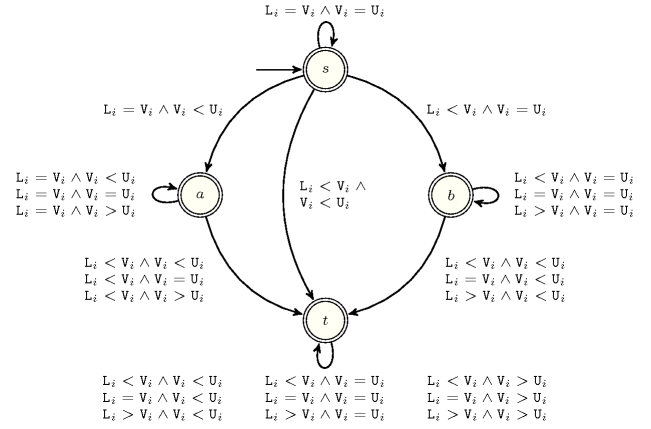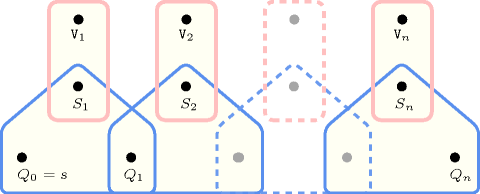## 5.222. lex_between

Origin
Constraint

$\mathrm{𝚕𝚎𝚡}_\mathrm{𝚋𝚎𝚝𝚠𝚎𝚎𝚗}\left(\mathrm{𝙻𝙾𝚆𝙴𝚁}_\mathrm{𝙱𝙾𝚄𝙽𝙳},\mathrm{𝚅𝙴𝙲𝚃𝙾𝚁},\mathrm{𝚄𝙿𝙿𝙴𝚁}_\mathrm{𝙱𝙾𝚄𝙽𝙳}\right)$

Synonym

$\mathrm{𝚋𝚎𝚝𝚠𝚎𝚎𝚗}$.

Arguments
 $\mathrm{𝙻𝙾𝚆𝙴𝚁}_\mathrm{𝙱𝙾𝚄𝙽𝙳}$ $\mathrm{𝚌𝚘𝚕𝚕𝚎𝚌𝚝𝚒𝚘𝚗}\left(\mathrm{𝚟𝚊𝚛}-\mathrm{𝚒𝚗𝚝}\right)$ $\mathrm{𝚅𝙴𝙲𝚃𝙾𝚁}$ $\mathrm{𝚌𝚘𝚕𝚕𝚎𝚌𝚝𝚒𝚘𝚗}\left(\mathrm{𝚟𝚊𝚛}-\mathrm{𝚍𝚟𝚊𝚛}\right)$ $\mathrm{𝚄𝙿𝙿𝙴𝚁}_\mathrm{𝙱𝙾𝚄𝙽𝙳}$ $\mathrm{𝚌𝚘𝚕𝚕𝚎𝚌𝚝𝚒𝚘𝚗}\left(\mathrm{𝚟𝚊𝚛}-\mathrm{𝚒𝚗𝚝}\right)$
Restrictions
 $\mathrm{𝚛𝚎𝚚𝚞𝚒𝚛𝚎𝚍}$$\left(\mathrm{𝙻𝙾𝚆𝙴𝚁}_\mathrm{𝙱𝙾𝚄𝙽𝙳},\mathrm{𝚟𝚊𝚛}\right)$ $\mathrm{𝚛𝚎𝚚𝚞𝚒𝚛𝚎𝚍}$$\left(\mathrm{𝚅𝙴𝙲𝚃𝙾𝚁},\mathrm{𝚟𝚊𝚛}\right)$ $\mathrm{𝚛𝚎𝚚𝚞𝚒𝚛𝚎𝚍}$$\left(\mathrm{𝚄𝙿𝙿𝙴𝚁}_\mathrm{𝙱𝙾𝚄𝙽𝙳},\mathrm{𝚟𝚊𝚛}\right)$ $|\mathrm{𝙻𝙾𝚆𝙴𝚁}_\mathrm{𝙱𝙾𝚄𝙽𝙳}|=|\mathrm{𝚅𝙴𝙲𝚃𝙾𝚁}|$ $|\mathrm{𝚄𝙿𝙿𝙴𝚁}_\mathrm{𝙱𝙾𝚄𝙽𝙳}|=|\mathrm{𝚅𝙴𝙲𝚃𝙾𝚁}|$ $\mathrm{𝚕𝚎𝚡}_\mathrm{𝚕𝚎𝚜𝚜𝚎𝚚}$$\left(\mathrm{𝙻𝙾𝚆𝙴𝚁}_\mathrm{𝙱𝙾𝚄𝙽𝙳},\mathrm{𝚅𝙴𝙲𝚃𝙾𝚁}\right)$ $\mathrm{𝚕𝚎𝚡}_\mathrm{𝚕𝚎𝚜𝚜𝚎𝚚}$$\left(\mathrm{𝚅𝙴𝙲𝚃𝙾𝚁},\mathrm{𝚄𝙿𝙿𝙴𝚁}_\mathrm{𝙱𝙾𝚄𝙽𝙳}\right)$
Purpose

The vector $\mathrm{𝚅𝙴𝙲𝚃𝙾𝚁}$ is lexicographically greater than or equal to the fixed vector $\mathrm{𝙻𝙾𝚆𝙴𝚁}_\mathrm{𝙱𝙾𝚄𝙽𝙳}$ and lexicographically smaller than or equal to the fixed vector $\mathrm{𝚄𝙿𝙿𝙴𝚁}_\mathrm{𝙱𝙾𝚄𝙽𝙳}$.

Example
$\left(〈5,2,3,9〉,〈5,2,6,2〉,〈5,2,6,3〉\right)$

The $\mathrm{𝚕𝚎𝚡}_\mathrm{𝚋𝚎𝚝𝚠𝚎𝚎𝚗}$ constraint holds since:

• The vector $\mathrm{𝚅𝙴𝙲𝚃𝙾𝚁}=〈5,2,6,2〉$ is greater than or equal to the vector $\mathrm{𝙻𝙾𝚆𝙴𝚁}_\mathrm{𝙱𝙾𝚄𝙽𝙳}=〈5,2,3,9〉$.

• The vector $\mathrm{𝚅𝙴𝙲𝚃𝙾𝚁}=〈5,2,6,2〉$ is less than or equal to the vector $\mathrm{𝚄𝙿𝙿𝙴𝚁}_\mathrm{𝙱𝙾𝚄𝙽𝙳}=〈5,2,6,3〉$.

Typical
 $|\mathrm{𝙻𝙾𝚆𝙴𝚁}_\mathrm{𝙱𝙾𝚄𝙽𝙳}|>1$ $\mathrm{𝚕𝚎𝚡}_\mathrm{𝚕𝚎𝚜𝚜𝚎𝚚}$$\left(\mathrm{𝙻𝙾𝚆𝙴𝚁}_\mathrm{𝙱𝙾𝚄𝙽𝙳},\mathrm{𝚄𝙿𝙿𝙴𝚁}_\mathrm{𝙱𝙾𝚄𝙽𝙳}\right)$
Symmetries
• $\mathrm{𝙻𝙾𝚆𝙴𝚁}_\mathrm{𝙱𝙾𝚄𝙽𝙳}.\mathrm{𝚟𝚊𝚛}$ can be decreased.

• $\mathrm{𝚄𝙿𝙿𝙴𝚁}_\mathrm{𝙱𝙾𝚄𝙽𝙳}.\mathrm{𝚟𝚊𝚛}$ can be increased.

Arg. properties

Suffix-contractible wrt. $\mathrm{𝙻𝙾𝚆𝙴𝚁}_\mathrm{𝙱𝙾𝚄𝙽𝙳}$, $\mathrm{𝚅𝙴𝙲𝚃𝙾𝚁}$ and $\mathrm{𝚄𝙿𝙿𝙴𝚁}_\mathrm{𝙱𝙾𝚄𝙽𝙳}$ (remove items from same position).

Usage

This constraint does usually not occur explicitly in practice. However it shows up indirectly in the context of the $\mathrm{𝚕𝚎𝚡}_\mathrm{𝚌𝚑𝚊𝚒𝚗}_\mathrm{𝚕𝚎𝚜𝚜}$ and the $\mathrm{𝚕𝚎𝚡}_\mathrm{𝚌𝚑𝚊𝚒𝚗}_\mathrm{𝚕𝚎𝚜𝚜𝚎𝚚}$ constraints: in order to have a complete filtering algorithm for the $\mathrm{𝚕𝚎𝚡}_\mathrm{𝚌𝚑𝚊𝚒𝚗}_\mathrm{𝚕𝚎𝚜𝚜}$ and the $\mathrm{𝚕𝚎𝚡}_\mathrm{𝚌𝚑𝚊𝚒𝚗}_\mathrm{𝚕𝚎𝚜𝚜𝚎𝚚}$ constraints one has to come up with a complete filtering algorithm for the $\mathrm{𝚕𝚎𝚡}_\mathrm{𝚋𝚎𝚝𝚠𝚎𝚎𝚗}$ constraint. The reason is that the $\mathrm{𝚕𝚎𝚡}_\mathrm{𝚌𝚑𝚊𝚒𝚗}_\mathrm{𝚕𝚎𝚜𝚜}$ as well as the $\mathrm{𝚕𝚎𝚡}_\mathrm{𝚌𝚑𝚊𝚒𝚗}_\mathrm{𝚕𝚎𝚜𝚜𝚎𝚚}$ constraints both compute feasible lower and upper bounds for each vector they mention. Therefore one ends up with a $\mathrm{𝚕𝚎𝚡}_\mathrm{𝚋𝚎𝚝𝚠𝚎𝚎𝚗}$ constraint for each vector of the $\mathrm{𝚕𝚎𝚡}_\mathrm{𝚌𝚑𝚊𝚒𝚗}_\mathrm{𝚕𝚎𝚜𝚜}$ and $\mathrm{𝚕𝚎𝚡}_\mathrm{𝚌𝚑𝚊𝚒𝚗}_\mathrm{𝚕𝚎𝚜𝚜𝚎𝚚}$ constraints.

Algorithm
Reformulation

The $\mathrm{𝚕𝚎𝚡}_\mathrm{𝚋𝚎𝚝𝚠𝚎𝚎𝚗}$$\left(\mathrm{𝙻𝙾𝚆𝙴𝚁}_\mathrm{𝙱𝙾𝚄𝙽𝙳},\mathrm{𝚅𝙴𝙲𝚃𝙾𝚁𝚂},\mathrm{𝚄𝙿𝙿𝙴𝚁}_\mathrm{𝙱𝙾𝚄𝙽𝙳}\right)$ constraint can be expressed as the conjunction $\mathrm{𝚕𝚎𝚡}_\mathrm{𝚕𝚎𝚜𝚜𝚎𝚚}$$\left(\mathrm{𝙻𝙾𝚆𝙴𝚁}_\mathrm{𝙱𝙾𝚄𝙽𝙳},\mathrm{𝚅𝙴𝙲𝚃𝙾𝚁𝚂}\right)\wedge$ $\mathrm{𝚕𝚎𝚡}_\mathrm{𝚕𝚎𝚜𝚜𝚎𝚚}$$\left(\mathrm{𝚅𝙴𝙲𝚃𝙾𝚁𝚂},\mathrm{𝚄𝙿𝙿𝙴𝚁}_\mathrm{𝙱𝙾𝚄𝙽𝙳}\right)$.

Systems
Keywords
Automaton

Figure 5.222.1 depicts the automaton associated with the $\mathrm{𝚕𝚎𝚡}_\mathrm{𝚋𝚎𝚝𝚠𝚎𝚎𝚗}$ constraint. Let ${𝙻}_{i}$, ${𝚅}_{i}$ and ${𝚄}_{i}$ respectively be the $\mathrm{𝚟𝚊𝚛}$ attributes of the ${i}^{th}$ items of the $\mathrm{𝙻𝙾𝚆𝙴𝚁}_\mathrm{𝙱𝙾𝚄𝙽𝙳}$, the $\mathrm{𝚅𝙴𝙲𝚃𝙾𝚁}$ and the $\mathrm{𝚄𝙿𝙿𝙴𝚁}_\mathrm{𝙱𝙾𝚄𝙽𝙳}$ collections. To each triple $\left({𝙻}_{i},{𝚅}_{i},{𝚄}_{i}\right)$ corresponds a signature variable ${S}_{i}$ as well as the following signature constraint:

$\left({𝙻}_{i}<{𝚅}_{i}\right)\wedge \left({𝚅}_{i}<{𝚄}_{i}\right)⇔{S}_{i}=0\wedge$

$\left({𝙻}_{i}<{𝚅}_{i}\right)\wedge \left({𝚅}_{i}={𝚄}_{i}\right)⇔{S}_{i}=1\wedge$

$\left({𝙻}_{i}<{𝚅}_{i}\right)\wedge \left({𝚅}_{i}>{𝚄}_{i}\right)⇔{S}_{i}=2\wedge$

$\left({𝙻}_{i}={𝚅}_{i}\right)\wedge \left({𝚅}_{i}<{𝚄}_{i}\right)⇔{S}_{i}=3\wedge$

$\left({𝙻}_{i}={𝚅}_{i}\right)\wedge \left({𝚅}_{i}={𝚄}_{i}\right)⇔{S}_{i}=4\wedge$

$\left({𝙻}_{i}={𝚅}_{i}\right)\wedge \left({𝚅}_{i}>{𝚄}_{i}\right)⇔{S}_{i}=5\wedge$

$\left({𝙻}_{i}>{𝚅}_{i}\right)\wedge \left({𝚅}_{i}<{𝚄}_{i}\right)⇔{S}_{i}=6\wedge$

$\left({𝙻}_{i}>{𝚅}_{i}\right)\wedge \left({𝚅}_{i}={𝚄}_{i}\right)⇔{S}_{i}=7\wedge$

$\left({𝙻}_{i}>{𝚅}_{i}\right)\wedge \left({𝚅}_{i}>{𝚄}_{i}\right)⇔{S}_{i}=8$.

##### Figure 5.222.1. Automaton of the $\mathrm{𝚕𝚎𝚡}_\mathrm{𝚋𝚎𝚝𝚠𝚎𝚎𝚗}$ constraint##### Figure 5.222.2. Hypergraph of the reformulation corresponding to the automaton of the $\mathrm{𝚕𝚎𝚡}_\mathrm{𝚋𝚎𝚝𝚠𝚎𝚎𝚗}$ constraint (since all states of the automaton are accepting there is no restriction on the last variable ${Q}_{n}$)